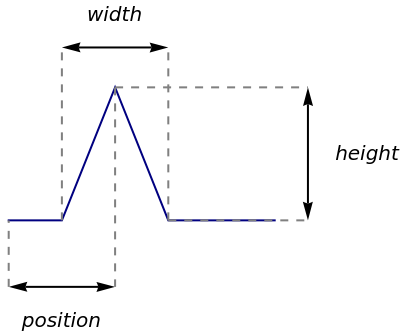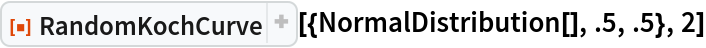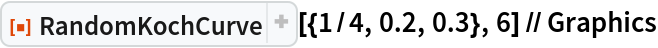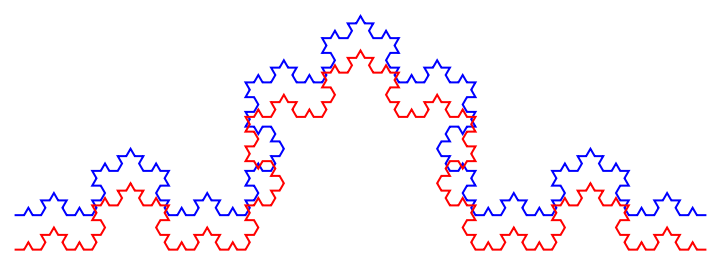Function Repository Resource:

# RandomKochCurve

Generate a random Koch curve

Contributed by: Sander Huisman
 ResourceFunction["RandomKochCurve"][{positiondist,widthdist,heightdist},n] makes a random Koch curve based on the distributions positiondist,widthdist and heightdist, for n iterations. ResourceFunction["RandomKochCurve"][{positiondist,widthdist,heightdist},{p1,p2,…},n] uses the starting points pi.

## Details

The position, width, and height specifications determine the geometry:Distributions can be any parametrized distribution such as UniformDistribution or NormalDistribution or can be a constant.
The three distributions are scaled from 0 to 1 to correspond to the length between the beginning and ending points.
ResourceFunction["RandomKochCurve"] returns a Line expression which can be visualized with Graphics.

## Examples

### Basic Examples (4)

Generate a Koch curve:

 In:=Out=Generate a random Koch curve and display it:

 In:=Out=Keep the position in the middle:

 In:=Out=Distributions don't have to be distributions but can be constant:

 In:=Out=### Scope (1)

 In:=Out=### Properties and Relations (1)

RandomKochCurve and KochCurve are related:

 In:=Out=### Neat Examples (1)

Create a forest:

 In:=Out=SHuisman

## Version History

• 1.0.0 – 28 June 2022